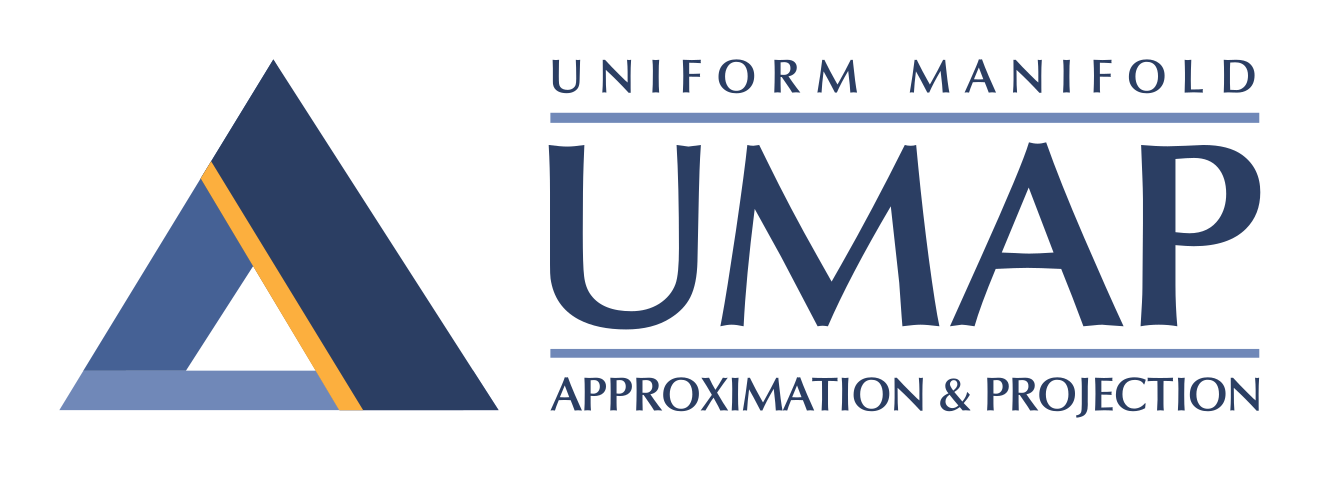# UMAP: Uniform Manifold Approximation and Projection for Dimension Reduction¶

Uniform Manifold Approximation and Projection (UMAP) is a dimension reduction technique that can be used for visualisation similarly to t-SNE, but also for general non-linear dimension reduction. The algorithm is founded on three assumptions about the data

1. The data is uniformly distributed on Riemannian manifold;

2. The Riemannian metric is locally constant (or can be approximated as such);

3. The manifold is locally connected.

From these assumptions it is possible to model the manifold with a fuzzy topological structure. The embedding is found by searching for a low dimensional projection of the data that has the closest possible equivalent fuzzy topological structure.

The details for the underlying mathematics can be found in our paper on ArXiv:

McInnes, L, Healy, J, UMAP: Uniform Manifold Approximation and Projection for Dimension Reduction, ArXiv e-prints 1802.03426, 2018

You can find the software on github.

Installation

Conda install, via the excellent work of the conda-forge team:

```conda install -c conda-forge umap-learn
```

The conda-forge packages are available for linux, OS X, and Windows 64 bit.

PyPI install, presuming you have numba and sklearn and all its requirements (numpy and scipy) installed:

```pip install umap-learn
```